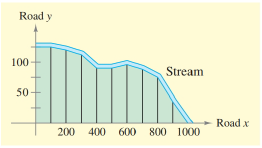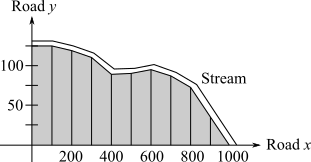Chapter 6.3, Problem 31E### Calculus: An Applied Approach (Min...

10th Edition
Ron Larson
ISBN: 9781305860919

#### Solutions

Chapter
Section### Calculus: An Applied Approach (Min...

10th Edition
Ron Larson
ISBN: 9781305860919
Textbook Problem
1 views

# Surveying In Exercises 31 and 32, use the Trapezoidal Rule to estimate the number of square feet of land in the lot, where x and y are measured in feet, as shown in the figure. The land is bounded by a stream and two straight roads. (The number of subintervals is shown in the figure.)x 0 100 200 300 400 500 y 125 125 120 112 90 90 x 600 700 800 900 1000 y 95 88 75 35 0 Spreadsheet at LarsonAppliedCalculus.com

To determine

To calculate: The number of square feet of land in the lot where the value of x and y get measured in feet with the use of Trapezoidal Rule according to the provided figure.

The provided figure of graph with subintervals is,And Provided table is,

x01002003004005006007008009001000y1251251201129090958875350

Explanation

Given Information:

The provided figure of graph with subintervals is,

And Provided table is,

x01002003004005006007008009001000y1251251201129090958875350

Formula used:

Trapezoidal Rule:

If a function f is continuous on [a,b], then

abf(x)dx(ba2n)[f(x0)+2f(x1)+...+2f(xn1)+f(xn)]

Calculation:

According to provided figure the graph is divided into 10 sections, so the value of n=10.

The minimum limit of x is 0 and maximum limit of x is 1000, so the value of a=0 and b=1000.

And y=f(x).

Calculation by Trapezoidal Rule:

for that get the end points of subintervals are,

f(x0)=125,f(x1)=125,f(x2)=120,f(x3)=112,f(x4)=90,f(x5

### Still sussing out bartleby?

Check out a sample textbook solution.

See a sample solution

#### The Solution to Your Study Problems

Bartleby provides explanations to thousands of textbook problems written by our experts, many with advanced degrees!

Get Started

#### Evaluate Evaluate the expression. 11. 21/281/2

Precalculus: Mathematics for Calculus (Standalone Book)

#### For Problems 11-18, evaluate each expression. (2.91)53.295

Mathematical Applications for the Management, Life, and Social Sciences